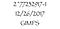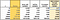# Code doodling: isPrimeLargest Mersenne Prime number

Sometimes I like to doodle and I often like to doodle with code.

Coding an isPrime function is a popular software engineering interview question. I’ve been on many hiring committees over the years and I’ve asked for code to determine if a number was prime many times. In a whiteboard interview, it’s common to ask for some code and then when the interviewee supplies something that works, ask them to make it more efficient. While asking for a function to determine if a number is prime is mathematical and I’m generally not hiring a mathematician, the concept of primes is so simple and fundamental that even if this is the first time you ever heard of a prime number, you can still understand what it is. Optimizing an isPrime algorithm does require some knowledge of math, but most coders can get through the “first try”. Working with primes opens the door to working through a process and making it more efficient. Making algorithms more efficient leads to questions of comparing algorithmic efficiency with Big(O).

A prime number is simply a number that is divisible only by itself and 1.

The image at the top of this article relates to the Great Internet Mersenne Prime Search. Mersenne primes are a subset of all primes that can be expressed as (2^n -1)where n is a whole positive integer. The largest n for which results in a Mersenne prime is number 77,232,917. It turns that the ancient concept of perfect numbers, formulated many years before Mersenne, is related to Mersenne primes.

The reason large primes are used in cryptography is because it is very expensive to compute the prime factors of large numbers so if you have a number that is the product of two very large prime numbers it will take a long time to find them. The public key in RSA encryption will be the product of two large primes and the private key will be the pair of primes. Even though factoring the multiple of large number is slow, multiplying large numbers is fast.

There are algorithms for prime factorization, prime generation and testing to see if a number is prime. Finding primes (prime generation) is often done with a sieve algorithm. The Sieve of Eratosthenes finds all primes in a range up to n with a time complexity of O(n log log n).

The fundamental theorem of arithmetic, aka the unique factorization theorem, states

every integer greater than 1 is a prime number or can be made by multiplying prime numbers together and that no matter how you get the primes it will always be the same set of primes

There are many sophisticated approaches for prime factorization including Shor’s algorithm which uses a quantum computer. If you google for code to test whether a number is prime you will find many answers.

I want to write a function that can determine if a number is prime. It may not be able to test for really large primes, but I want to make the algorithm’s Big O as good as it can be — that is, I want to eliminate as much iteration and intermediary computation as possible. I can also run my code on a computer with time tests to see if my Big(O) analyses predict better execution times.

I’m going to use JavaScript but I’m not going to use any of the optimized array functionality to test my times. I will take advantage of multiplication over division, as multiplication can be faster on a computer than division, and I can avoid checking the divisor for 0.

I’m writing the code as close to pseudocode as possible. I’ll work through five algorithms and determine their Big O. Then I’ll run the code on my laptop and time the results for each algorithm testing for primes in the ranges 1–10¹, 1–10², 1–10³, 1–10⁴, 1–10⁵, and 1–10⁶.

# Algorithms

Intuition about primes (knowing some math helps here):

• a number is not a prime if it is divisible by any number besides 1 and itself
• any number can be expressed as the product of factors prime numbers
• an even number besides 2 cannot be prime because it would be divisible by 2
• a number expressed as a product of factors cannot have a factor greater than the value of its own square root
• the square root of a whole positive number is generally less than or equal to 1/2 the number (exceptions are 2 and 3)

Because I’m timing my algorithms using the JavaScript `console.time` command, and running a series of ranges, I am automatically adding an extra iteration for the set of ranges. I won’t include that iteration through the range of numbers tested in stating the Big O for the algorithm.

To start I will just iterate through all values in a range and test to see which are prime by dividing each number by every number in the range until a primes is found or none is found indicating a prime was found. In the worst case, when the number is prime the algorithm is Big O(n). In reality the number of iterations is less then n because when a number is determined to be not prime the iteration stops and there are more non-prime numbers than prime numbers.

`function isPrime(n) {  for (let i = 2; i < n; i++) {    if (n % i == 0)      return false;  }  return true;}console.time("check");for (let range = 1; range < 7; range++) {  let n = Math.pow(10,range);  for (let i = 1; i < n; i++) {    let prime = isPrime(i);  }}console.time("check");`

Finding that sieves can be used to determine primes, the second algorithm will create a sieve a prime numbers that can be used to test numbers with successively greater values against numbers found to be prime. The idea is to start with small primes and then check each subsequent number to see if it is divisible by these primes and therefore not prime itself.

This algorithm involves capturing primes in a data structure so there is a cost in space for using a sieve. The algorithm is optimized by seeding the sieve with 2 and 3, the lowest value primes and then only test odd numbers. The Big(O) for this algorithm is n*n/2 because we only test 1/2 the numbers in the range by excluding even numbers, but we test an ever growing list of captured primes. In fact, the Big O is a less the n²/2 because the number of captured primes is far less the quantity of numbers tested.

`const sieve = [2, 3];function findPrimes(n) {  //test odd numbers only  for (let i = 5; i <= n; i += 2) {    let prime = true;    //check every new number against divisibility by existing primes    for (let j = 0; j <= sieve.length; j++) {      if (i % sieve[j] === 0) {        prime = false;      }    }    if (prime) sieve.push(i);  }}for (let range = 1; range < 7; range++) {  let n = Math.pow(10, range);  console.log("range", n)  console.time("check");  findPrimes(n);  // console.log(i, "Prime 1", isPrime(i))  console.timeEnd("check");}3. Half the Numbers`

In this algorithm, I abandon the sieve but focus on the looking for factors of the number tested for prime that are less then 1/2 the number. In my reasoning I state that in general 1/2 the value of a number is greater than the square root of the number and that the square root of a number is the largest possible factor of a number besides the number itself.

This is not the most efficient algorithm because I’m looking at possible factors greater than the largest possible factor but it is more efficient than the “first try” which looks at all numbers between 2 and the number tested.

My loop termination test is 2 * i < n instead of i < n/2 because I want to use multiplication instead of division.

For this code Big(O) = n/2.

`function isPrime(n) {  for (let i = 2; 2 * i < n; i++) {    if (n % i == 0)      return false;  }  return true;}for (let range = 1; range < 4; range++) {  let n = Math.pow(10, range);  console.time("check");  for (let i = 1; i < n; i++) {    let p = isPrime(i);  }  console.timeEnd("check");}`

In this method I only iterate to the square root of the number tested but I test from both ends of the range in a single iteration thereby cutting the number of iterations in half. For this algorithm the Big(O) = 1/2 * log(n).

In this algorithm we find the log value and then perform two tests and two counter updates per iteration which reduces the number of iterations but increases the number of operations at each step of the iteration. I’m also using the square root function for lack of a way around it.

`function isPrime(n) {  let top = Math.ceil(Math.sqrt(n));  let bottom = 2;  while (top >= bottom) {    if ((n % top === 0) || (n % bottom === 0)) {      return false;    }    top = top - 1;    bottom = bottom + 1;  }  return true;}for (let range = 1; range < 4; range++) {  let n = Math.pow(10, range);  console.time("check");  for (let i = 1; i < n; i++) {    let p = isPrime(i);  }  console.timeEnd("check");}`

In this algorithm I sensibly narrow the iteration down to numbers between 2 and the square root of the number tested. I also exclude all even numbers from the. For this algorithm Big(O)=1/2 * log(n).

In this algorithm we only test from 3 to the value of the square root of n, but we don’t iterate through odd numbers. So even though the Big(O) is the same for both algorithms 4 and 5 the actual work performed is significantly different.

I’m using i * i ≤ n instead of i ≤ n^(1/2) to provide maximum speed in operations.

`function isPrime(n) {  //check if n is a multiple of 2 - exclude even numbers  if (n !== 2 && n % 2 == 0) return false;  //if not, then just check the odds up to square root  for (let i = 3; i * i <= n; i += 2) {    console.log(i)    if (n % i == 0)      return false;  }  return true;}for (let range = 1; range < 4; range++) {  let n = Math.pow(10, range);  console.time("check");  for (let i = 1; i < n; i++) {    let p = isPrime(i);  }  console.timeEnd("check");}`

# Results

The results of running the isPrime on ranges 10¹ through 10⁶ are shown below. Algorithms 1,2 and 3 perform significantly worse than algorithms 4 and 5, and 5 outperforms all. These timings are not that scientific as there are a lot of variables that go into how many milliseconds it actually takes to run an algorithm on data, but they are repeatable on the machine I ran them on.Time to determine “is prime” (ms) for each algorithm tested

In conclusion the Big(O) does help predict the actual time it takes to run the algorithm, but similar Big(O) can be off in timing by a factor of 10. In looking at the numbers there are a few anomalies: timings for a value of n=100 are always less than for n=10 and, while the overall 10⁷ performance for algorithm 5 is the best, its performance when n=10 is not as good as first try.

In an interview situation, I’d be happy with the first try and if I did want to push for a more efficient algorithm, I’d probably provide some of the intuitions that could give rise to an algorithm with a better Big(O).

Software/Web Engineer and Instructor

## More from Rebecca Peltz

Software/Web Engineer and Instructor